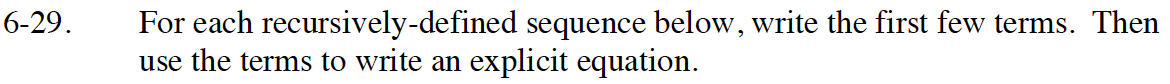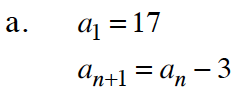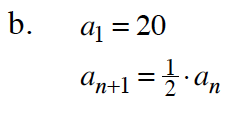Home > GB8I > Chapter cc46 > Lesson cc46.1.3 > Problem6-29

6-29.
1. For each recursively-defined sequence below, write the first few terms. Then use the terms to write an explicit equation. Homework Help ✎

1. a1 = 17
an+1 = an – 3

2. a1 = 20
an+1 =· anFor help, write out a sequence:
a1 = 17, a2 = 14, and so on.

an = 20 − 3na1 = 20, a2 = 10, and so on.